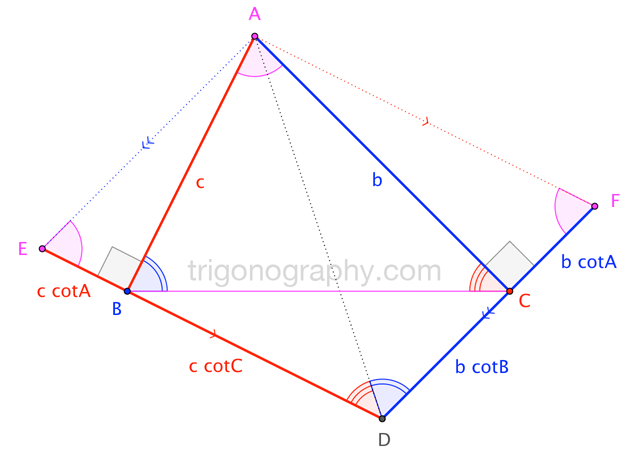## A Cotangent Identity for Triangles$$|\overline{AB}|\cdot|\overline{DE}| = |EADF| = |\overline{AC}|\cdot|\overline{DF}| \qquad\to$$

$$\large c^2\,\left(\, \cot A + \cot C \,\right) \;=\; b^2\,\left(\, \cot A + \cot B \,\right)$$